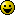# Parallax tutorial by VIB (Read 11204 times)

Started by Master Yoda, May 18, 2010, 03:19:13 AM
Share this topic:

#### Master Yoda##### Parallax tutorial by VIB
#1  May 18, 2010, 03:19:13 AM
••hi guys!Max beta was looking for this tutorial, luckily I took time but I find it!
The VIB page is offline now and before it is lost, i add the tutorial here!

Quote
first take a look at this picture:notice the blue line, that is your z-offset (char line) .. the floor sprite is between the red lines .. A is the distance between the top and the char line .. B is the distance between char line and bottom .. h is the sprite height (this is here just for reference, note that h=A+B)

your z-offset should be = 240 - B (240 is mugen screen height) .. and you should get the top delta of the floor from the emulator by calculating "between how much the top of the floor moves" / "how much a standing character moved" ..

then you calculate the xscale using:
xscale = 1, x
x = ((1-d)*B/A+1)/d
where A is the distance between the top and the char line
B is the distance between char line and bottom
d is the sprite delta, x parameter

in chunli's stage for example .. h = 64, A = 41, B = 23, zoffset = 217, d = .765
so it is like this:
delta = .765,1
xscale = 1, 1.479
; x = ((1-.765)*23/41+1)/.765

then, sprites in the top should have delta = floor's delta (.765 in chunli's) .. sprites in the bottom should have delta = (floor's delta * x) .. .765*1.479= 1.131 in chunli's stage

the characters won't slide and you won't have to adjust zoffset because the xscale was calculated in order to make that the delta in the char line to be = 1 (that line of the floor will move in the same speed as the characters)

you can see the final delta values in this picture to understanding it
notice that the deltas are proportional in the same ratio .. remember Tales ?? =Ptop delta = .765 * 1 = .765
char delta = 1 .. this is the reason of all the calculation, to keep this = to 1
bottom delta = .765 * 1.479 = 1.131

got it ?? the z-offset you get from the emulator, and then you calculate the bottom delta so that the char delta is equal to 1 ..

#### XamΣeta##### Re: Parallax tutorial by VIB
#2  May 18, 2010, 04:14:36 PMYAY!I guess its back to the old drawing board for me now.
"We need other people in order to create the circumstances for the learning that we are here to generate" RIP Adam Yauch aka MCA
www.instagram.com/tanooki_ninja